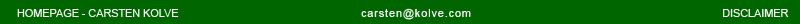MAYA, TUTORIALS, ROLLING ELLIPSE

This tutorial shows you how to roll an ellipse on the floor, using Maya expressions. Have no fear, I won't go too much into mathematical details here, but will provide you with ready to use expressions for copy and paste. If you really want to dive into the wonders of elliptical calculus, you'll find the links to sites I used to obtain the mathematical background information at the end of this tutorial. I'll assume you are familiar with basic Maya workflow (expression editing, geometry creation, etc.) and some basic math, your angular unit should be "degrees".

DEFINITIONS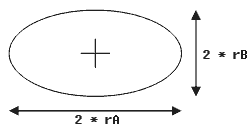I guess you know what an ellipse is, so you are already familiar with the fact, that you need two radii to construct one: I will call them rA and rB here.

THE ROLLING CIRCLE
Before we'll roll an ellipse, let's see how to roll a circle as we can apply the insights gained from this on the ellipse proplem.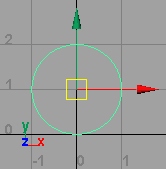Create a nurbsCircle (radius = 1.0; axis = z;), rename it to "circle", switch to othographic front view, move the center of the circle to [0,1]. Note: Instead of a nurbsCircle you can also create a nurbsCylinder, so you'll be able to watch the movement of the object in perspective view more clearly.

 \$pi = 3.141592; // radius of circle \$rA = 1.0; // get translation in x direction \$x = circle.translateX; // perimeter of circle \$per = 2.0 * \$pi *\$rA; // rotation circle has made when travelling x units \$rot = -360.0 * (\$x / \$per); // apply rotation to circle circle.rotateZ = \$rot;

What have we just done? When you move a circle on the ground, the length of the arc has to equal the length travelled in x-direction. So the rotation required to do this can be obtained as a function of the complete rotation of a circle (360°), the length travelled in x-direction and the perimeter of the circle. A final multiplication with -1 will ensure the circle rotates in the correct direction.

Alternativly you can use this expression, which is a condensed form of the upper one, faster to execute but not very readable

 \$rA = 1.0; circle.rotateZ = -57.29578 * circle.translateX / \$rA;

Move the circle in x direction and see how the expression rotates your circle correctly.

THE ROLLING ELLIPSE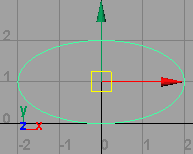Now on to the rolling ellipse: Scale the circle by 2.0 in x-direction, rename it to "ellipse".

We will start like we did with the circle and find an expression for the perimeter of the ellipse. Unfortunatly there is no simple formula for the circumference of an ellipse like the one we used for the circle (2*PI*r). In fact, obtaining the exact result is not an opportunity here as it would require us to deal with sums of infinitely many terms, elliptic integrals and the likes... everything not only very complicated and difficult to implement but also very computational expensive. Luckily there exist quite a lot of much simpler approximations for the perimeter of an ellipse, so we just use these. It's in the nature of approximation that it inherits a relative error, but we will choose formulas that will have a very small error so the effect won't be very visible. I will give you some approximation formulas, sorted by relative error (from big to small), choose one wich you works best for you (in many cases already the simplest one will give good results.

 \$pi = 3.141592; \$rA = 2.0; \$rB = 1.0; // compute perimeter // // simple \$per = \$pi*(\$rA+\$rB); // Kepler \$per = 2.0*\$pi*sqrt(\$rA*\$rB); // Euler \$per = \$pi*sqrt(2.0*(\$rA*\$rA+\$rB*\$rB)); // unknown \$per = \$pi*sqrt(2.0*(\$rA*\$rA+\$rB*\$rB)-(pow((\$rA-\$rB),2)*0.5)); // Ramanujan I \$per = \$pi*(3*(\$rA+\$rB)-sqrt((3*\$rA+\$rB)*(\$rA+3*\$rB))); // Ramanujan II \$h = pow((\$rA-\$rB),2)/(pow((\$rA+\$rB),2)); \$per = \$pi*(\$rA+\$rB)*(1+3*\$h/(10+sqrt(4-3*\$h)));

Now that we have got the perimeter, let's compute the angle of rotation required to fit the translation of the ellipse, just like we did with the circle (note that we are working in radians here, hence the 2*PI instead of 360°):

 \$x = ellipse.translateX; \$rot = -2*\$pi*\$x/\$per; ellipse.rotateZ = rad_to_deg(\$rot);

IMPORTANT: This is mathematically wrong! I made the assumption that the ellipse had everywhere the same curvature (like a circle), but this is not true. So this will only look correct if you do not have very elongated ellipses. I will update this tutorial when I have found a simple solution to this problem (the arc length and angle relationship is not linear!).

Now that we have found the rotation, we still need to adjust the y-position of the ellipse's center.

 \$y = abs((-sin(\$rot)*tan(\$rot)*\$rA*\$rA)-(cos(\$rot)*\$rB*\$rB))/      sqrt(\$rA*\$rA*pow(tan(\$rot),2)+\$rB*\$rB); ellipse.translateY = \$y;

Yes, that looks scary. I won't go into much detail about this term, but basically it epresses the y-component of the point of tangency for a specific angle, rotational transformed to fit the current coordinate system for the ellipse. Add the expression to your scene, move the ellipse in x-direction and watch it rolling on the floor.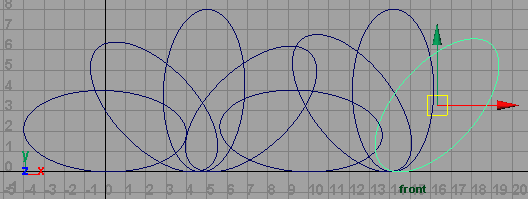Enjoy :)